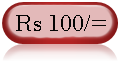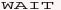Web Development : Starting from Concept, HTML, CSS, Web Site Develpment to Website Launching.
Written by: Sanjay Srivastava
B.Tech (Honours);IIT Kharagpur
PMP ; PMI USA

Class - VIII - Maths:- INDEX

1. Squares & Square Roots
2. Cubes & Cube Roots
4. Profit Loss & Discount
5. Compound Interest
6. Algebraic Identities7. Polynomials8. Linear Equations9. Parallel Lines10. Types of Quadrilaterals11. Construction of Quadrilaterals12. Circles13. Areas14. Surface Areas15. Volumes16. StatisticsClass - VIII - Maths

## What is a Cube Root (Page 3/7)

In a Perfect Cube the all the numbers of factors should have pair of three.

Question - Type 2 - Is 200 is a perfect cube ?

Answer - 2- Make the factors

200= 2*2*2*5*5

Since 5*5 are only two pair, therefore 200 is not a perfect cube.

Question - Type 3 - With what number 200 is to be multiplied to make it perfect cube

Answer - 3- Make the factors

200= 2*2*2*5*5

Since 5*5 are only two pair, therefore 200 must be multiplied with 5 to make it a perfect cube.

i.e. 200*5=1000=10*10*10
Now this is a perfect cube and the cube root is 10.